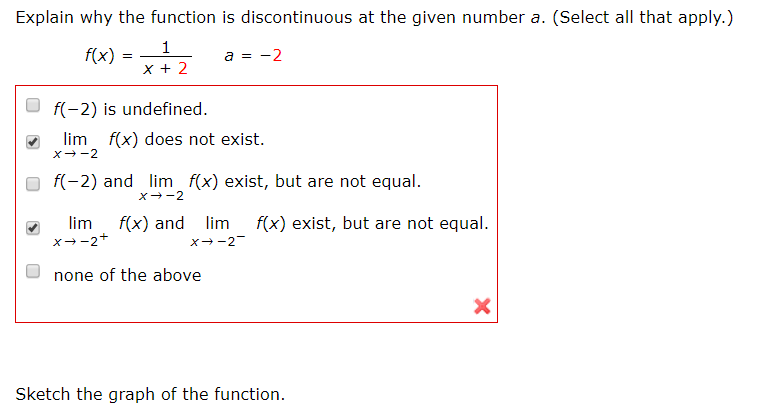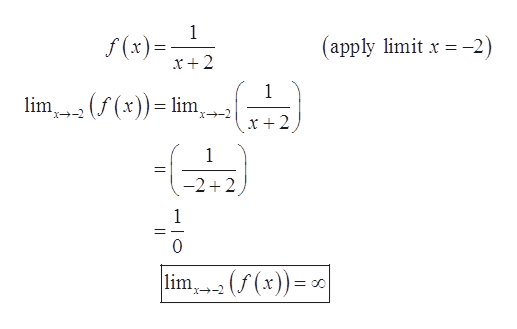# Explain why the function is discontinuous at the given number a. (Select all that apply.)f(x) =a = -2f(-2) is undefined.limf(x) does not exist.f(-2) and lim f(x) exist, but are not equal.limx--2+f(x) and limf(x) exist, but are not equal.X--2-none of the aboveSketch the graph of the function.

Question
16 viewshelp_outlineImage TranscriptioncloseExplain why the function is discontinuous at the given number a. (Select all that apply.) f(x) = a = -2 f(-2) is undefined. lim f(x) does not exist. f(-2) and lim f(x) exist, but are not equal. lim x--2+ f(x) and lim f(x) exist, but are not equal. X--2- none of the above Sketch the graph of the function. fullscreen
check_circle

Step 1

To explain the discontinuity of the given function at the point a= -2.

Step 2

Given information:

The function is given below.

Step 3

Calculation:

Solve the given fu...help_outlineImage Transcriptionclosef (x)= x+2 (apply limit x = -2) lim2 (f (x))= lim, x + 2 -2+2 lim2 (f (x)) = 0 =00 x+-2 fullscreen

### Want to see the full answer?

See Solution

#### Want to see this answer and more?

Solutions are written by subject experts who are available 24/7. Questions are typically answered within 1 hour.*

See Solution
*Response times may vary by subject and question.
Tagged in

### Continuity